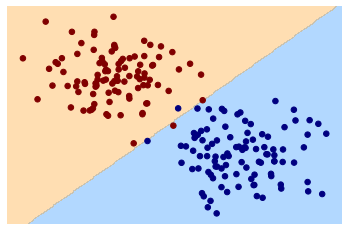# Mlf-Machine Learning Concepts

Last updated: April 9th, 2020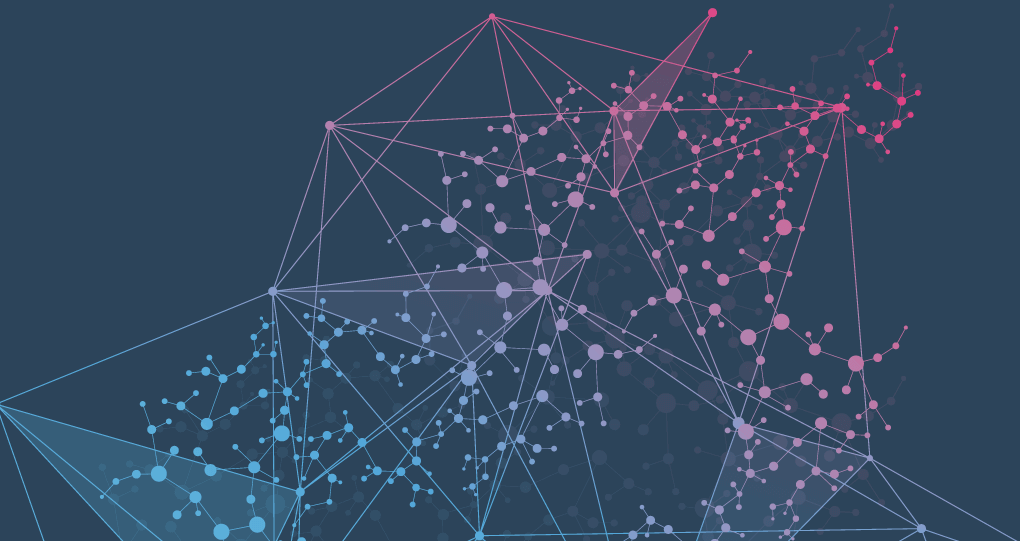# Machine Learning concepts¶

The primary goal of most of the machine learning algorithm is to construct a model. In this lesson we will discuses about important concepts covered to better understand how to use these tools from one of the field's best practitioners and teachers.## What is learning?¶

There are several definitions of learning. One of the simplest definitions is “The activity or process of gaining knowledge or skill by studying, practicing, being taught, or experiencing something”. Similar to various definitions available of learning, there are various categories of learning methods.

As a human, we learn a lot of things during our entire life. Some of them are on based the based of that assumption our experience and some of them are based on memorization. On the basis of that we can divide learning methods into five parts:

1. Rote Learning (memorization): Memorizing things without knowing the concept/ logic behind them
2. Passive Learning (instructions): Learning from a teacher/expert.
3. Analogy (experience): Learning new things from our past experience.
4. Inductive Learning (experience): On the basis of past experience formulating a generalized concept.
5. Deductive Learning: Deriving new facts from past facts.## What is concept learning?¶

In terms of machine learning, the concept learning can be formulated as “Problem of searching through a predefined space of potential hypotheses for the hypothesis that best fits the training examples”-Tom Michell.

For example, to identify different vehicles among all the vehicles based on some specific set of features defined over a large set of features. This special set of features differentiates the subset of cars in the set of vehicles. This set of features that differentiate cars, can be called a concept.## Instance, features and Labels¶

Let's explore fundamental machine learning terminology.

• Target: The target is whatever the output of the input variables. It could be the individual classes that the input variables maybe mapped to in case of a classification problem or the output value range in a regression problem. If the training set is considered then the target is the training output values that will be considered.

• Feature: Features are individual independent variables that act as the input in your system. Prediction models use features to make predictions. More simply, you can consider one column of your data set to be one feature. Sometimes these are also called attributes. And the number of features are called dimensions.

• Labels: Labels are the final output. You can also consider the output classes to be the labels. The label could be the future price of wheat, the kind of animal shown in a picture, the meaning of an audio clip, or just about anything.

• Instance: An example is a particular instance of data, x.

A large part of machine learning consists of go through data, process them to a shape / form that makes sense, and pass that into the model to train. So let's review the main components of a DataFrame:

• the index labels (these are the bold numbers from 0 to 9 on the left hand side of the table)
• the column names (these are the bold names on the top of the table)
• the data itself (this is everything else inside the actual cells of the table)

In this context, we could identify the column names as the features and each rows as instances.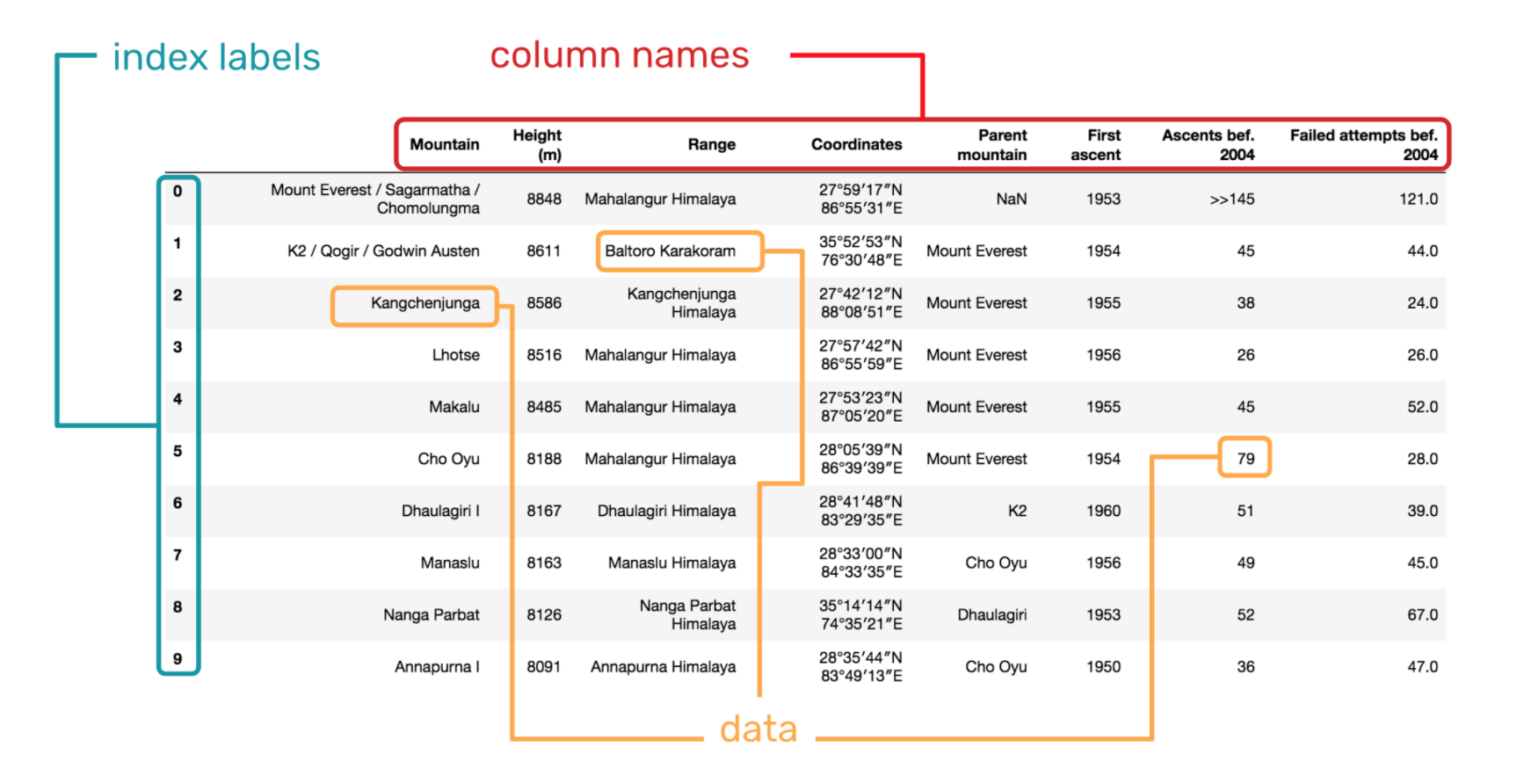## Hypothesis representation and Space¶

The discussion of hypotheses in machine learning can be confusing for a beginner, especially when hypotheses has a distinct, but related meaning in statistics (e.g. statistical hypothesis testing) and more broadly in science (e.g. scientific hypothesis)

Machine learning, specifically supervised learning, can be described as the desire to use available data to learn a function that best maps inputs to outputs.

Technically, this is a problem called function approximation, where we are approximating an unknown target function (that we assume exists) that can best map inputs to outputs on all possible observations from the problem domain.

An example of a model that approximates the target function and performs mappings of inputs to outputs is called a hypothesis in machine learning.

The choice and the configuration of the algorithm define the space of possible hypothesis that the model may represent.

A common notation is used where lowercase-h (h) represents a given specific hypothesis and uppercase-h (H) represents the hypothesis space that is being searched.

• h (hypothesis): A single hypothesis, e.g. an instance or specific candidate model that maps inputs to outputs and can be evaluated and used to make predictions.

• H (hypothesis set):The Hypothesis space H is the set all the possible models h which can be learned by the current learning algorithm

The choice of algorithm and algorithm configuration involves choosing a hypothesis space that is believed to contain a hypothesis that is a good or best approximation for the target function. This is very challenging, and it is often more efficient to spot-check a range of different hypothesis spaces.

#### Graphical Example¶

We have n samples(instance).

• Each point have two coordinates: (x, y)(Feature).
• Each point have a color: label.
• Our objective is to find a function (hypothesis) that approximate the target function:

h(x, y) → color

In :
import pandas as pd
import numpy as np
import seaborn as sns
import matplotlib.pyplot as plt
sns.set()

In :
from sklearn.datasets import make_blobs

X, y = make_blobs(n_samples=200, centers=2,
random_state=0, cluster_std=1.3)

In :
plt.scatter(X[:, 0], X[:, 1], c=y, s=50, cmap='summer')
plt.xlabel('Feature x1')
plt.ylabel('Feature x2')
plt.show()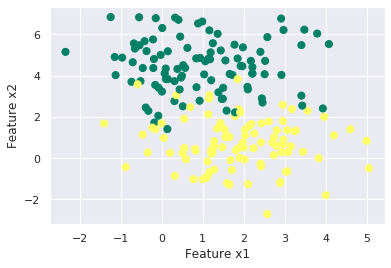First Idea:

• h(x, y) = yellow if x < k

      if not green
• H = possible values < k, color >

• Algorithm: search < k, yellow > That best separate both colors.

In :
plt.scatter(X[:, 0], X[:, 1], c=y, s=50, cmap='summer')
plt.axhline(y=2, xmin=-2, xmax=5)
plt.xlabel('Feature x1')
plt.ylabel('Feature x2')
plt.show()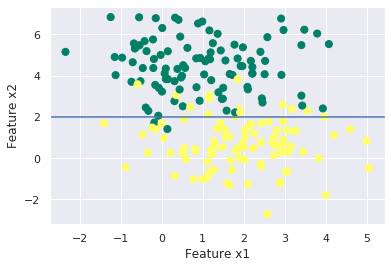## The Inductive Learning Hypothesis¶

Any hypothesis found to approximate the target function that well cover a sufficiently large set of training examples will also approximate the target function well over any other unobserved examples.

Assumptions for Inductive Learning Algorithms:

• The training sample represents the population
• The input features permit discrimination

This means that the model tries to induce a general rule from a set of observed instances

#### Inductive Bias¶

In machine learning, the term Inductive Bias refers to a set of (explicit or implicit) assumptions made by a learning algorithm in order to perform induction, that is, to generalize a finite set of observation (training data) into a general model of the domain. Without a bias of that kind, induction would not be possible, since the observations can normally be generalized in many ways. Treating all these possibilities equally, i.e., without any bias in the sense of a preference for specific types of generalization (reflecting background knowledge about the target function to be learned), predictions for new situations could not be made.

This means that Inductive Bias (also known as learning bias) of a learning algorithm is the set of assumptions that the learner uses to predict outputs given inputs that it has not encountered. In machine learning, it aims to construct algorithms that are able to learn to predict a certain target output.

Examples:

• vertical or horizontal lines.
In :
centers = [[1, -1], [-1, 1]]
X, y = make_blobs(n_samples=200, centers=centers, cluster_std=0.6,random_state=0)

In :
plt.scatter(X[:, 1], X[:, 0], c=y, s=50, cmap='summer')
plt.axhline(y=0, xmin=-2, xmax=5)
plt.xlabel('Feature x1')
plt.ylabel('Feature x2')
plt.show()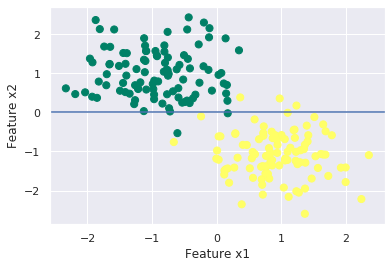• lines
In :
plt.scatter(X[:, 1], X[:, 0], c=y, s=50, cmap='summer')

ydata = [_*2 for _ in X[:, 1]]

plt.plot(X[:, 1], ydata, 'b')

plt.xlabel('Feature x1')
plt.ylabel('Feature x2')
plt.show()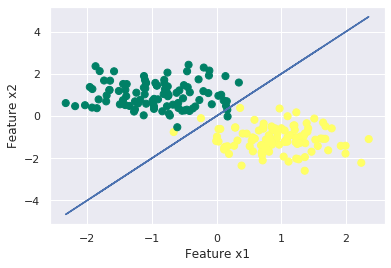• Parabola
In :
# create 1000 equally spaced points between -10 and 10
x = np.linspace(-2, 2, 500)
# calculate the y value for each element of the x vector
yy = x**2 + 2*x - 0.5
plt.scatter(X[:, 1], X[:, 0], c=y, s=50, cmap='summer')
plt.plot(x, yy, 'b')
plt.xlabel('Feature x1')
plt.ylabel('Feature x2')
plt.ylim((4,-4))
plt.xlim((4,-4))
plt.show()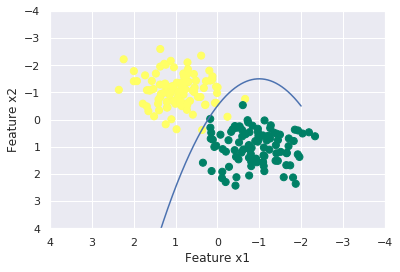###### Bias-Variance Trade-off – Will discuss in more detail in model selection and evaluation¶## What is Decision Boundary?¶

Decision boundary helps to differentiate probabilities into positive and negative class. In classification problem with two classes, a decision boundary or decision surface is a hypersurface that partitions the underlying vector space into two sets, one for each class.

Let´s define a function to visualize the decision bounderies of a classification

In :
def visualize_classifier(model, X, y, ax=None, cmap='jet'):
ax = ax or plt.gca()

# Plot the training points
ax.scatter(X[:, 0], X[:, 1], c=y, s=30, cmap=cmap,
clim=(y.min(), y.max()), zorder=3)
ax.axis('tight')
ax.axis('off')
xlim = ax.get_xlim()
ylim = ax.get_ylim()

xx, yy = np.meshgrid(np.linspace(*xlim, num=200),
np.linspace(*ylim, num=200))
Z = model.predict(np.c_[xx.ravel(), yy.ravel()]).reshape(xx.shape)

# Create a color plot with the results
n_classes = len(np.unique(y))
contours = ax.contourf(xx, yy, Z, alpha=0.3,
levels=np.arange(n_classes + 1) - 0.5,
cmap=cmap, clim=(y.min(), y.max()),
zorder=1)

ax.set(xlim=xlim, ylim=ylim)


We will define a linear model

In :
# create the linear model classifier
from sklearn.linear_model import SGDClassifier
clf = SGDClassifier()
# fit (train) the classifier
clf.fit(X, y)

Out:
SGDClassifier(alpha=0.0001, average=False, class_weight=None,
early_stopping=False, epsilon=0.1, eta0=0.0, fit_intercept=True,
l1_ratio=0.15, learning_rate='optimal', loss='hinge',
max_iter=1000, n_iter_no_change=5, n_jobs=None, penalty='l2',
power_t=0.5, random_state=None, shuffle=True, tol=0.001,
validation_fraction=0.1, verbose=0, warm_start=False)

Now we can visualize the decision boundaries

In :
visualize_classifier(clf,X, y)

<ipython-input-18-db72ddeaea70>:18: UserWarning: The following kwargs were not used by contour: 'clim'
contours = ax.contourf(xx, yy, Z, alpha=0.3,